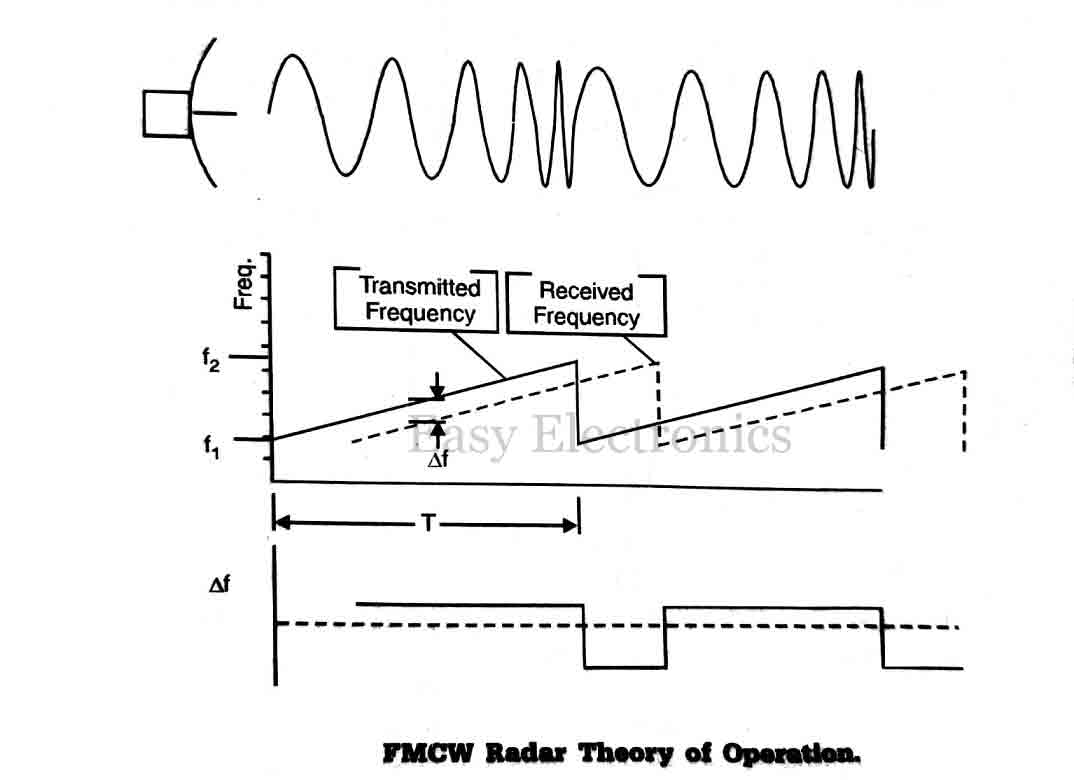In this lecture, we are going to learn about the frequency modulated CW radar, where we will know about the basic principle, theory of operation, some equations regarding the FMCW radar and application of frequency modulated CW radar.

Before starting this lecture we will know what is the drawback of CW radar.

## What is the drawback of CW radar?

• The transmitter in CW radar is not modulated. Therefore it can neither provide a range of the target nor sense which particular cycle of oscillation is being received at any instant. This is the major drawback of the CW radar.

This major drawback can be eliminated by frequency modulating the transmitted signal even it increases the bandwidth.

The spectrum of a CA transmission can be broadened by the application of modulation, amplitude, frequency, or phase.

An example of amplitude modulation is pulsed radar.

## Block diagram of Frequency Modulated CW Radar:

A block diagram illustrating the principle of FMCW radar is shown in the below figure.A portion of the transmitter signal acts as the reference signal required to produce the beat frequency. It introduces directly into the received via cable.

Ideally, the isolation between transmitting and receiving antenna is made sufficiently large so as to reduce to a negligible level the transmitter leakage, which arrives at the receiver via coupling between the antennas.

The beat frequency is amplified and limited to remove any amplitude fluctuations.

The frequency of amplitude limited beat note is usually measured with a cycle-counting frequency calibrated in distance.

## Operation of Frequency Modulated CW radar:

A widely used technique to broadening the spectrum of CW radar is to frequency modulate the carrier signal.

The timing mark is the changing frequency.  The transit time is proportional to the difference in the frequency between the echo signal and transmitter signal.The greater the transmitter frequency deviation in a given time interval, the more accurate the measurement of the transit time, and the greater will be the transmitted spectrum.

By measuring the frequency of the return signal, the time delay between transmission and reception can be measure and therefore the range determined as before.

The simplest way to modulate the wave is to linearly increase the frequency. in other words, the transmitted frequency will change at a constant rate.

The FMCW radar system measures the instantaneous difference between the transmitted and received frequencies, Δf. This difference is directly proportional to the time delay, Δt, which takes the radar signal to reach the target and return.

From this, the range can be found using the formula,

R = c \times \frac{Δt}{2} ….. Eq.1

The time delay can be found as follows:

\boxed{Δt = T \times \frac{Δf}{(f_2 - f_1)}}…..Eq.2

Where,

• f_2=Maximum frequency
• f_1= minimum frequency
• T= period of sweep from f1 to f2,
• Δf= the difference between transmitted and received frequencies

There is a slight problem, which occurs when the sweep resets the frequency, and the frequency difference becomes negative (as shown in the plot of Δf vs time).

The system uses a discriminator to clip off the negative signal, leaving only the positive part, which is directly proportional to the range.

Combining the above two Eq.1 and Eq.2 into a single form for the range can be written by,

\boxed{R = \frac{2cTΔf}{(f_2 - f_1)}} …..Eq.3

Another way to construct an FMCW radar system is to compare the phase difference between the transmitted and received signals after they have been demodulated to receiver the sweep information. This system does not have to discriminate the negative values of Δf. In either case, however, the maximum unambiguous range will still be determined by the period, namely

R_{unamb}= c \times \frac{T}{2}

The rate of change of the frequency is given by,

(\frac{\text{d}f}{\text{d}t})=\frac{2Δf}{T/2}

where,

• Δf=The range of frequency
• T=Modulation period

The beat frequency f_b is corresponding to the range (R) may be given by,

[\frac{\text{d}f}{\text{d}t}][\frac{2R}{c}]=[\frac{2Δf}{T/2}][\frac{2R}{c}]

\boxed{f_b=\frac{8R.4Δf}{f_m}}…..Eq.4

where fm= minimum beat frequency

\boxed{R_{min}=\frac{c}{8Δf}}…..Eq.5

In case of altimeter R_{min} is the fixed error in the range.

## Application of Frequency Modulated CW Radar:

One of the major applications of the FMCW radar principle has been an altimeter onboard aircraft to measure height above the ground. The large target cross-section and the relatively short-range required of altimeter permit low transmitter power and low antenna gain. Since the relative motion, and hence the doppler velocity between the aircraft and ground, is small the effect of the doppler frequency shift may usually be neglected.Hello friends, my name is Trupal Bhavsar, I am the Writer and Founder of this blog. I am Electronics Engineer(2014 pass out), Currently working as Junior Telecom Officer(B.S.N.L.) also I do Project Development, PCB designing and Teaching of Electronics Subjects.

This site uses Akismet to reduce spam. Learn how your comment data is processed.Join Our Telegram Group!